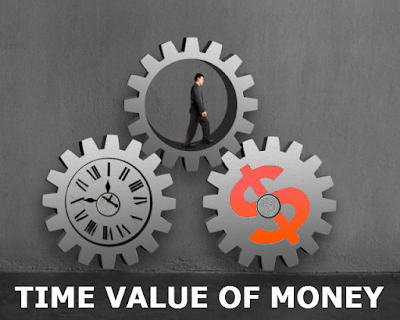In financial management, time value of money is a very important concept. It is useful for comparing investment alternatives and to solve pr...

In financial management, time value of money is a very important concept. It is useful for comparing investment alternatives and to solve problems involving loans, mortgages, leases, savings, and annuities.

The time value of money (TVM) is the idea that money available at the present time is worth more than the same or equal amount in the future due to its potential and good earning capacity.The time value of money give the great benefit of receiving money now than the later.

It is founded on time preference.

The principle of the time value of money explains the payment and earning of interest. Interest, whether it is on a bank deposit or debt.

It is also useful for investment. An investor is willing to spend their money now if they expect a favorable return on their investment.

EXAMPLE:

If you would choose to receive the 10,000 now. After all, five years is a long time to wait. Why would any person defer payment into the future when he or she could have the same amount of money now? For most of us, taking the money in current time is just basic instinctive. So at the most basic level, the time value of money demonstrates that, all things being equal, it is good to have money now than the later.

But why is this? Payment of Rs.100 has the same value as Rs.100 one year from now, doesn't it? Actually, although the payment is the same, you can use it if you have the money now because over time you can make and earn more interest on your money.

Back to our example: by receiving 10,000 today, you want to increase the future value of your money by investing and gaining interest over a period of time. For Option B, you don't have time, and the payment received in five years would be your future value. Here, we understand by example:

If you are choosing Option A, your future value will be 10,000 plus any interest gaining over the five years. The future value for Option B, on the other hand, would only be 10,000. So how can you calculate exactly how much more Option A is worth, compared to Option B? Assume interest rate is 5%.

Future value of investment:
=P X (1+i) n

•First year:
= (10000 x 0.05) +10000
=10500

•Second year:
=10000 x (1+0.50)1+1
=22500

•Third year:
=10000 x (1+0.50)1+1+1
=33750

•Fourth year:
=10000 x (1+0.50)1+1+1+1
=50625

FEW COMMON TERMS USED TO CALCULATE TIMES VALUE OF MONEY:

You can do calculation of the fifth value if you are given any four of: Interest Rate, Number of Periods, Payments, Present Value, and Future Value.  All of these factors for value is briefly defined as under.

Interest is a charge of borrowed money, normally stated as a percentage of the amount borrowed for a specific period of time. Simple interest is computed only on the original borrowed amount. It is the return on that amount for one time period. Compound interest can be calculated as per each period on the original amount borrowed plus all unpaid interest.

Number of Periods:
Periods are intervals of time. They are intentionally not stated in years since each interval must comport to a compounding period for a single amount or a payment period for an income.

Payments:
Payments are a series of cash flows. Payments must represent all outflows and inflows, outflows represent the negative amount and inflows represent the positive amount.

Present Value:
Single Amount
Annuity

Present Value is an amount today that is equivalent to a future payment that has been discounted by an applicable interest rate.  The future amount can be a single sum that will be received at the end of the last period, as a series of equal time period payments (an annuity), or both.  Since money has time value, the present value of a promised future amount is worth less the longer you have to wait to receive it.

PV    =    FV
(1+I) n

Future Value:
Single Amount
Annuity

Future Value is the amount of money that an investment with a fixed amount, compounded interest rate will grow to by some future date. The investment can be a single sum deposited at the beginning of the first period, a series of equally-spaced payments (an annuity), or both.  Since money has time value, we always expect the future value to be greater than the present value.

A method for pay back a loan in equal installments. Part of each payment goes toward interest and any remainder is used to reduce the principal. As the balance of the loan is reduced, a progressively larger portion of each payment goes toward reducing principal.

Cash Flow Diagram:
A cash flow diagram is a picture of a financial problem that shows all cash inflows and outflows during the time. It can help you to visualize a problem and to determine if it can be solved by TVM methods.Name

ltr
item
Vyas Infotech: TIMES VALUE OF MONEY: CONCEPT, FORMULA & EXAMPLES
TIMES VALUE OF MONEY: CONCEPT, FORMULA & EXAMPLES
https://3.bp.blogspot.com/-vl-s9okKPe8/VuwBVZtAidI/AAAAAAAAAGk/YU0Oy57Sq3YHTPsIqvTIfGi3lGEPfMh2A/s400/time%2Bvalue%2Bof%2Bmoney.png
https://3.bp.blogspot.com/-vl-s9okKPe8/VuwBVZtAidI/AAAAAAAAAGk/YU0Oy57Sq3YHTPsIqvTIfGi3lGEPfMh2A/s72-c/time%2Bvalue%2Bof%2Bmoney.png
Vyas Infotech
https://www.vyasinfotech.com/2016/03/times-value-of-money-concept-formula_28.html
https://www.vyasinfotech.com/
https://www.vyasinfotech.com/
https://www.vyasinfotech.com/2016/03/times-value-of-money-concept-formula_28.html
true
5236136159781716145
UTF-8